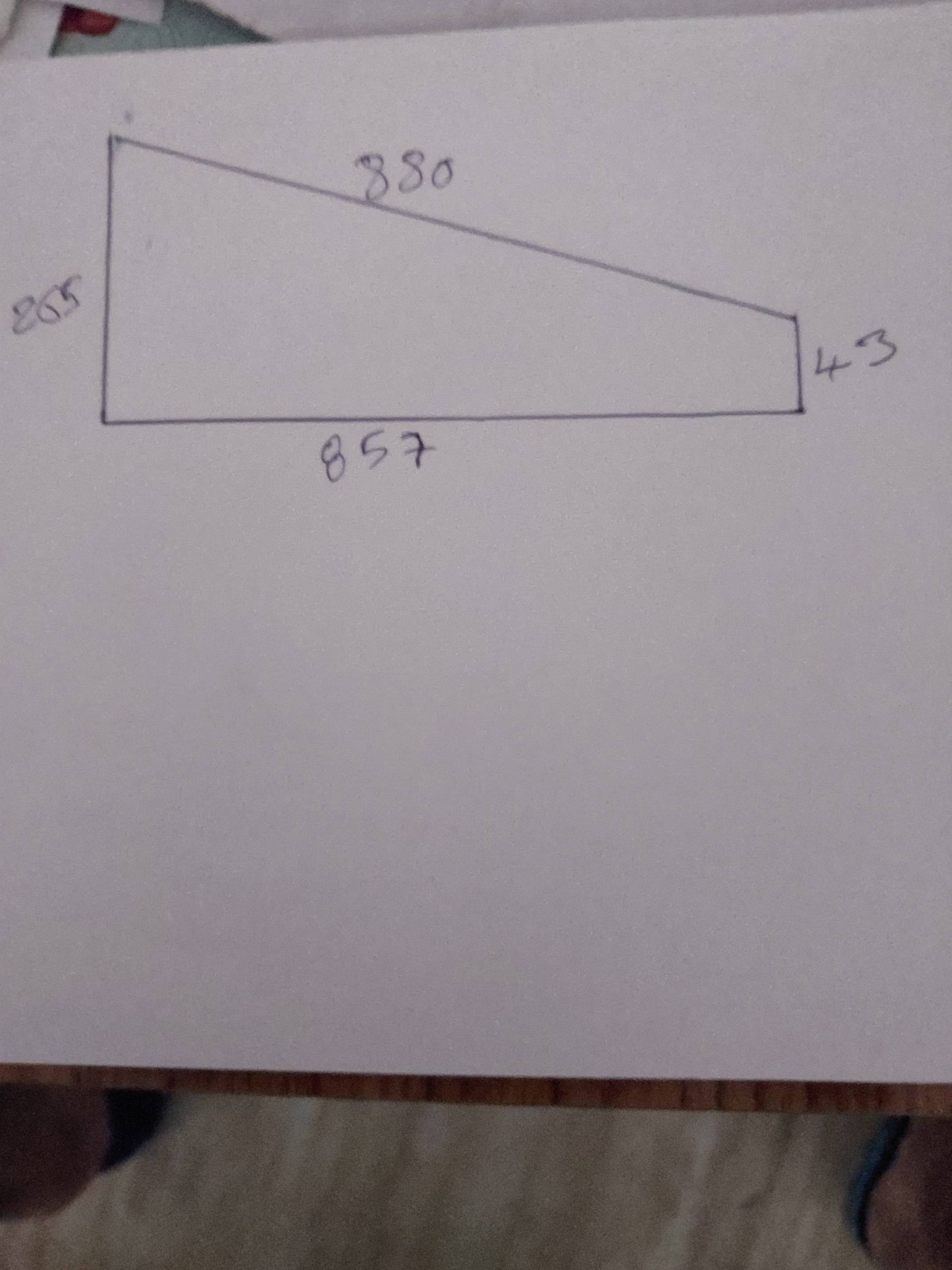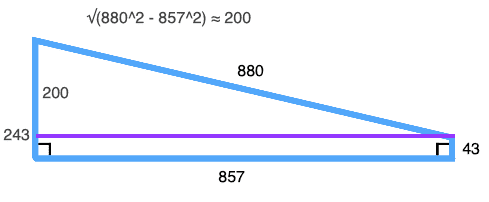# How to find the area of this irregular quadrilateral?

• MHB
• Srinivas
In summary, the conversation discusses the difficulty in finding the area of an irregular quadrilateral using only the lengths of its sides. The suggested method is to divide the quadrilateral into triangles and use Heron's formula, but this requires knowledge of at least one angle. Another formula, Bretschneider's formula, can be used but also requires knowledge of two opposite angles. The possibility of the given dimensions being measured on a sphere is also mentioned. Ultimately, it is determined that there is not enough information to solve the problem with just the side lengths.

#### SrinivasLast edited by a moderator:
Srinivas said:
https://www.physicsforums.com/attachments/11884
How irregular is this? The picture makes it look like a right trapezoid, which has a known formula.

There may be a way to get the area of a quadrilateral using only the sides (like Heron's formula for triangles) but the only process I'm sure of requires knowledge of one of the angles as well. The usual method for finding the area of an irregular figure is to break it into triangles and find the sum of the areas of the triangles. If you have the angle of just one of these triangles you can find the length of the diagonal and can use Heron's formula to find the area of the triangle. Then you can get the other two angles and work from there.

-Dan

topsquark said:
How irregular is this? The picture makes it look like a right trapezoid, which has a known formula.

There may be a way to get the area of a quadrilateral using only the sides (like Heron's formula for triangles) but the only process I'm sure of requires knowledge of one of the angles as well. The usual method for finding the area of an irregular figure is to break it into triangles and find the sum of the areas of the triangles. If you have the angle of just one of these triangles you can find the length of the diagonal and can use Heron's formula to find the area of the triangle. Then you can get the other two angles and work from there.

-Dan
Two right angles.

Dan, two right angles are there in the image.

Srinivas said:
Dan, two right angles are there in the image.
Okay, so this is not an irregular quadrilateral, it's a right trapezoid. The area for a trapezoid is $A = \dfrac{1}{2} (b_1 + b_2) h$. What can you use for the bases and the height?

-Dan

•berkeman
Srinivas said:
Dan, two right angles are there in the image.
It wasn't clear from the drawing that the angles at the base were right angles.

In any case, if you have an irregular quadrilateral you can find its area using Bretschneider's Formula - https://en.wikipedia.org/wiki/Bretschneider's_formula. However, in addition to the lengths of the four sides, you also need to know the measures of two opposite angles.

•topsquark
I think the first thing to do in this problem would be to determine if such a figure can be created with two right angles with the given dimensions.243 ≠ 265

Perhaps the lengths were measured on a sphere?

•robphy
Srinivas said:
Dan, two right angles are there in the image.
If I'm not mistaken, the quadrilateral in the picture cannot exist. Could you double check the numerical values of the problem, please? Just to make sure, we're talking about the right problem.

I think you don't have enough information to solve the problem from just the side lengths.

You can decompose the figure into two triangles. However, all you know about each triangle are the lengths of two of its sides. You need an additional piece of information - either the length of the third side (ie one of the diaongals of the quadrilateral) or an angle - in order to solve those triangles and so determine their areas.